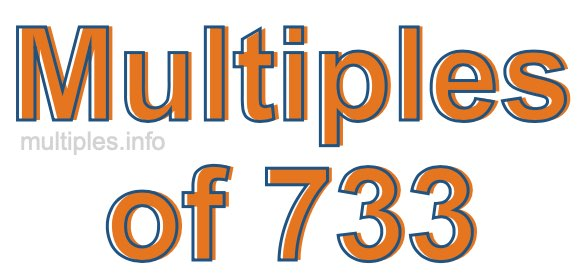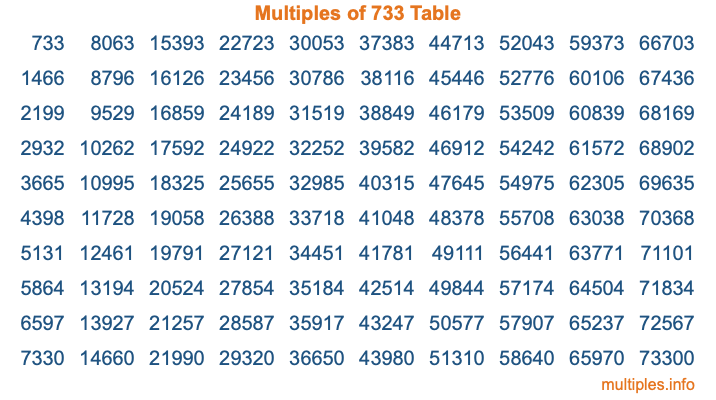Multiples of 733Welcome to the Multiples of 733 page. Here we will first teach you everything you will ever need to know about the multiples of 733, and then give you a study guide summary of everything we taught you to make sure you remember it all. Use this page to look up facts and learn information about the multiples of 733. This page will make you a multiples of seven hundred thirty-three expert!

Definition of Multiples of 733
Multiples of 733 are all the numbers that when divided by 733 equal an integer. Each of the multiples of 733 are called a multiple. A multiple of 733 is created by multiplying 733 by an integer.

Therefore, to create a list of multiples of 733, you start with 1 multiplied by 733, then 2 multiplied by 733, then 3 multiplied by 733, and so on for as long as you want. Thus, the list of the first five multiples of 733 is 733, 1466, 2199, 2932, and 3665. To see a larger list of multiples of 733, see the printable image of Multiples of 733 further down on this page. We also have a category where you can choose any nth multiple of 733.

Multiples of 733 Checker
The Multiples of 733 Checker below checks to see if any number of your choice is a multiple of 733. In other words, it checks to see if there is any number (integer) that when multiplied by 733 will equal your number. To do that, we divide your number by 733. If the the quotient is an integer, then your number is a multiple of 733.

Is  a multiple of 733?

Least Common Multiple of 733 and ...
A Least Common Multiple (LCM) is the lowest multiple that two or more numbers have in common. This is also called the smallest common multiple or lowest common multiple and is useful to know when you are adding our subtracting fractions. Enter one or more numbers below (733 is already entered) to find the LCM.

Check out our LCM Calculator if you need more details about the Least Common Multiple or if you need the LCM for different numbers for adding and subtraction fractions.

nth Multiple of 733
As we stated above, 733 is the first multiple of 733, 1466 is the second multiple of 733, 2199 is the third multiple of 733, and so on. Enter a number below to find the nth multiple of 733.

th multiple of 733

Multiples of 733 vs Factors of 733
733 is a multiple of 733 and a factor of 733, but that is where the similarities end. All postive multiples of 733 are 733 or greater than 733. All positive factors of 733 are 733 or less than 733.

Below is the beginning list of multiples of 733 and the factors of 733 so you can compare:

Multiples of 733: 733, 1466, 2199, 2932, 3665, etc.

Factors of 733: 1, 733

As you can see, the multiples of 733 are all the numbers that you can divide by 733 to get a whole number. The factors of 733, on the other hand, are all the whole numbers that you can multiply by another whole number to get 733.

It's also interesting to note that if a number (x) is a factor of 733, then 733 will also be a multiple of that number (x).

Multiples of 733 vs Divisors of 733
The divisors of 733 are all the integers that 733 can be divided by evenly. Below is a list of the divisors of 733.

Divisors of 733: 1, 733

The interesting thing to note here is that if you take any multiple of 733 and divide it by a divisor of 733, you will see that the quotient is an integer.

Multiples of 733 Table
Below is an image of the first 100 multiples of 733 in a table. The table is in chronological order, column by column. The first column has the first ten multiples of 733, the second column has the next ten multiples of 733, and so on.The Multiples of 733 Table is also referred to as the 733 Times Table or Times Table of 733. You are welcome to print out our table for your studies.

Negative Multiples of 733
Although not often discussed or needed in math, it is worth mentioning that you can make a list of negative multiples of 733 by multiplying 733 by -1, then by -2, then by -3, and so on, to get the following list of negative multiples of 733:

-733, -1466, -2199, -2932, -3665, etc.

Multiples of 733 Summary
Below is a summary of important Multiples of 733 facts that we have discussed on this page. To retain the knowledge on this page, we recommend that you read through the summary and explain to yourself or a study partner why they hold true.

There are an infinite number of multiples of 733.

A multiple of 733 divided by 733 will equal a whole number.

733 divided by a factor of 733 equals a divisor of 733.

The nth multiple of 733 is n times 733.

The largest factor of 733 is equal to the first positive multiple of 733.

733 is a multiple of every factor of 733.

733 is a multiple of 733.

A multiple of 733 divided by a divisor of 733 equals an integer.

733 divided by a divisor of 733 equals a factor of 733.

Any integer times 733 will equal a multiple of 733.

Multiples of a Number
Here you can get the multiples of another number, all with the same attention to detail as we did for multiples of 733 on this page.

Multiples of
Multiples of 734
Did you find our page about multiples of seven hundred thirty-three educational? Do you want more knowledge? Check out the multiples of the next number on our list!